# Mag-fields-electromag-induc.ppt

1. Jun 2023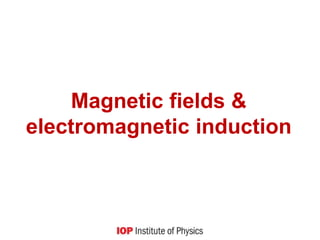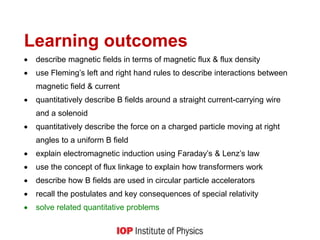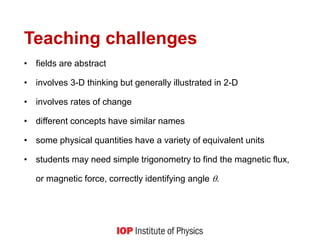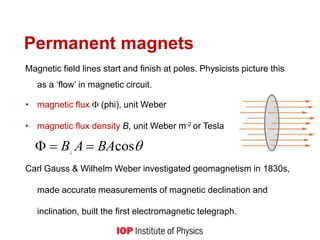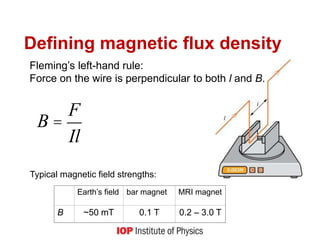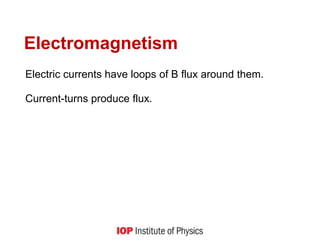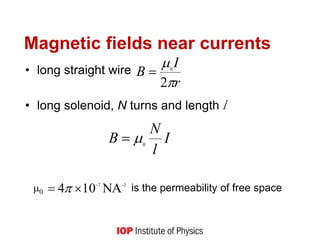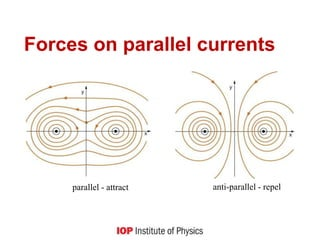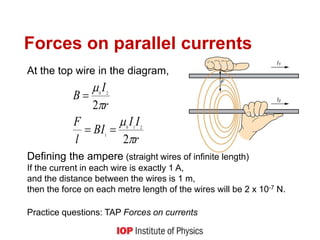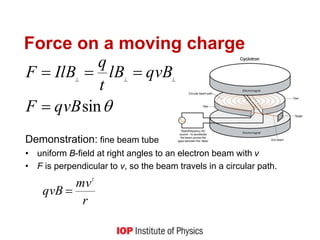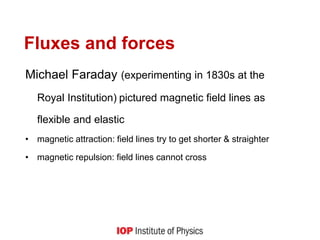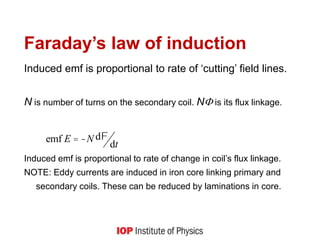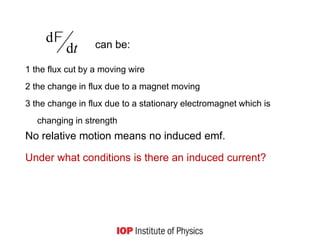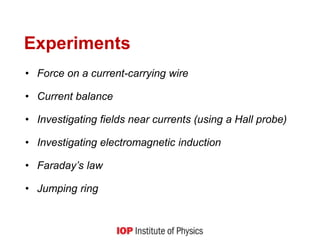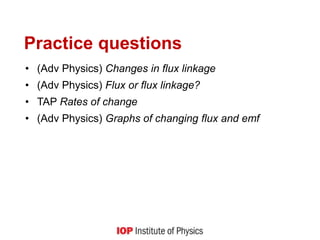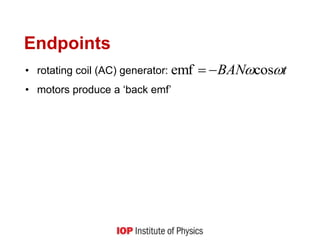1 von 16

### Mag-fields-electromag-induc.ppt

• 2. Learning outcomes  describe magnetic fields in terms of magnetic flux & flux density  use Fleming’s left and right hand rules to describe interactions between magnetic field & current  quantitatively describe B fields around a straight current-carrying wire and a solenoid  quantitatively describe the force on a charged particle moving at right angles to a uniform B field  explain electromagnetic induction using Faraday’s & Lenz’s law  use the concept of flux linkage to explain how transformers work  describe how B fields are used in circular particle accelerators  recall the postulates and key consequences of special relativity  solve related quantitative problems
• 3. Teaching challenges • fields are abstract • involves 3-D thinking but generally illustrated in 2-D • involves rates of change • different concepts have similar names • some physical quantities have a variety of equivalent units • students may need simple trigonometry to find the magnetic flux, or magnetic force, correctly identifying angle .
• 4. Permanent magnets Magnetic field lines start and finish at poles. Physicists picture this as a ‘flow’ in magnetic circuit. • magnetic flux (phi), unit Weber • magnetic flux density B, unit Weber m-2 or Tesla Carl Gauss & Wilhelm Weber investigated geomagnetism in 1830s, made accurate measurements of magnetic declination and inclination, built the first electromagnetic telegraph.  cos BA A B    
• 5. Defining magnetic flux density B = F Il Typical magnetic field strengths: Earth’s field bar magnet MRI magnet B ~50 mT 0.1 T 0.2 – 3.0 T Fleming’s left-hand rule: Force on the wire is perpendicular to both l and B.
• 6. Electromagnetism Electric currents have loops of B flux around them. Current-turns produce flux.
• 7. Magnetic fields near currents • long straight wire • long solenoid, N turns and length l  is the permeability of free space r I B   2 0  I l N B 0   -2 7 NA 10 4    
• 8. Forces on parallel currents parallel - attract anti-parallel - repel
• 9. Forces on parallel currents r I I BI l F r I B     2 2 2 1 0 1 2 0    At the top wire in the diagram, Defining the ampere (straight wires of infinite length) If the current in each wire is exactly 1 A, and the distance between the wires is 1 m, then the force on each metre length of the wires will be 2 x 10-7 N. Practice questions: TAP Forces on currents
• 10. Demonstration: fine beam tube • uniform B-field at right angles to an electron beam with v • F is perpendicular to v, so the beam travels in a circular path. Force on a moving charge  sin qvB F qvB lB t q IlB F        r mv qvB 2 
• 11. Fluxes and forces Michael Faraday (experimenting in 1830s at the Royal Institution) pictured magnetic field lines as flexible and elastic • magnetic attraction: field lines try to get shorter & straighter • magnetic repulsion: field lines cannot cross
• 12. Faraday’s law of induction Induced emf is proportional to rate of ‘cutting’ field lines. N is number of turns on the secondary coil. N is its flux linkage. Induced emf is proportional to rate of change in coil’s flux linkage. NOTE: Eddy currents are induced in iron core linking primary and secondary coils. These can be reduced by laminations in core. emf E = -N dF dt
• 13. 1 the flux cut by a moving wire 2 the change in flux due to a magnet moving 3 the change in flux due to a stationary electromagnet which is changing in strength No relative motion means no induced emf. Under what conditions is there an induced current? dF dt can be:
• 14. Experiments • Force on a current-carrying wire • Current balance • Investigating fields near currents (using a Hall probe) • Investigating electromagnetic induction • Faraday’s law • Jumping ring
• 15. Practice questions • (Adv Physics) Changes in flux linkage • (Adv Physics) Flux or flux linkage? • TAP Rates of change • (Adv Physics) Graphs of changing flux and emf
• 16. Endpoints • rotating coil (AC) generator: • motors produce a ‘back emf’ t BAN  cos emf  